﻿ Radiative Effect on MHD Fluid Flow in a Vertical Channel under Optically Thick Approximation

### Radiative Effect on MHD Fluid Flow in a Vertical Channel under Optically Thick Approximation

M. O Ibrahim, K. K. Asogwa, I. J Uwanta, B. G Dan ShehuOPEN ACCESSPEER-REVIEWED

## Radiative Effect on MHD Fluid Flow in a Vertical Channel under Optically Thick Approximation

M. O Ibrahim1, K. K. Asogwa2,, I. J Uwanta3, B. G Dan Shehu4

1Department of Mathematics, University of Ilorin, Kwara State, Nigeria

2Department of Physical and Computer Sciences, College of Natural and Applied Science, McPherson University, Abeokuta. Ogun State, Nigeria

3Department of Mathematics, Usmanu Danfodiyo University, Sokoto, Nigeria

4Sokoto Energy Research Centre, Usmanu Danfodiyo University, Sokoto, Nigeria

### Abstract

Many non linear problems that arise in real life situations defy analytical solution; hence numerical techniques are desirable to find the solution of such equations. In this study we use the Newton scheme method from Taylor series to solve fourth order non linear problem. The velocity profiles and temperature profile are studied for different physical parameters like, Magnetic parameter M, Radiation F and thermal Grashof number Ga. The results obtained after computation taking into cognizance of the parameters present shows that the Magnetic parameter M increases with increasing velocity, while the trend reverses with radiation and thermal Grashof number in a vertical channel under optically thick approximation. It also observed that temperature rise with increasing values of ai and τ2 and trend of heat transfer coefficient due to thermal conduction h increases with the rate of change of .

### At a glance: Figures

12
Prev Next

• Ibrahim, M. O, et al. "Radiative Effect on MHD Fluid Flow in a Vertical Channel under Optically Thick Approximation." American Journal of Modeling and Optimization 1.3 (2013): 36-42.
• Ibrahim, M. O. , Asogwa, K. K. , Uwanta, I. J. , & Shehu, B. G. D. (2013). Radiative Effect on MHD Fluid Flow in a Vertical Channel under Optically Thick Approximation. American Journal of Modeling and Optimization, 1(3), 36-42.
• Ibrahim, M. O, K. K. Asogwa, I. J Uwanta, and B. G Dan Shehu. "Radiative Effect on MHD Fluid Flow in a Vertical Channel under Optically Thick Approximation." American Journal of Modeling and Optimization 1, no. 3 (2013): 36-42.

 Import into BibTeX Import into EndNote Import into RefMan Import into RefWorks

### 1. Introduction

The different models and approximations of the optical transfer operator are employed in multidimensional problems of radiative correction for remote sensing of ocean, cloudiness, in theories of light and image propagation in turbine media, and in theoretical and computational fundamentals of optical-electronic remote sensing systems. Boundary-value problem of radiation transfer in optically thick finite planar layers is considered which are represented, in particular, by: cloudiness, outbreaks of aerosol particles, dust plumes which are generated under influence of vast fires (forest, peat, steppe, anthropogenic), and the atmosphere with optically thick layers of trace gases.

The study of flow of electrically conducting fluid, the so-called magneto-hydrodynamics (MHD) has a lot of attention due to its diverse applications. In astrophysics and geophysics, it is applied to the study of stellar and solar structures, interstellar matter, and radio propagation through the ionosphere. In engineering, it finds its application in MHD pumps, MHD bearings, nuclear reactors, geothermal energy extraction and in boundary layer control in the field of aerodynamics. A survey of MHD studies could be found in Crammer and Pai ; Moreau . For example, Bharti et al  Steady flow of power-law fluids across a circular cylinder; while Kearsley  studied problem of steady state Couette flow with viscous heating. Makinde and Osalusi  considered a MHD steady flow in a channel with slip at permeable boundaries. Abel et al.  analyzed the effect of the buoyancy force and thermal radiation in MHD boundary layer viscoelastic fluid flow over a continuously moving stretching surface.

More recently, many researchers have focused attention on MHD applications where the operating temperatures are high. For example, at high temperatures attained in some engineering devices, gas can be ionized and so become electrically conducting. The ionized gas or plasma can be made to interact with the magnetic field and alter the heat and friction characteristics of the system. It is important to study the effect of the interaction of magnetic field on the temperature distribution and heat transfer when the fluid is not only electrically conducting but also when it is capable of emitting and absorbing thermal radiation. Heat transfer by thermal radiation is important when we are concerned with space technology applications and in power engineering. Thus, Grief et al.  obtained an exact solution for the problem of laminar convective flow in a vertical heated channel within the optically thin limit of Cogley et al. . Makinde and Mhone  investigated the effect of thermal radiation on MHD oscillatory flow in a channel filled with saturated porous medium and non-uniform wall temperatures. Recently Taiwo and Ogunlaran  studied Numerical solution of fourth order linear ordinary differential equations by cubic spline collocation tau method.

This paper, is an extension of the work of Ibrahim  to include magnetic field. Hence it is proposed to study radiation effect on MHD fluid flow in a vertical channel under optically thick approximation.

### 2. Formulation of the Problem

The physical model above shows a stream of radiating flow velocity u flowing over the plate. The radiating flow is at its temperature T. the temperature of the wall. The gravity effect on the radiation and the laminar convection of the gas are also taken into account, the gravity effect is denoted by g.

Consider the fully developed laminar flow between parallel walls as shown in the above physical model i.e. the velocity will be a maximum at the center and zero at the walls, also the velocity distribution will be symmetric about theaxis i.e.. With the width of the channel being equal to. Since in the assumption, the temperatures of the walls are the same and are maintained at a constant temperature gradient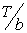so that the wall temperatureis given by. Where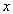is the coordinate in the vertical direction,

Here The problem of radiation effect on MHD fluid flow in a vertical channel under optically thick approximation. formulated, analysed and solved numerically. The- axis is taken along the plate in the vertically upward direction and also the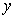-axis is taken normal to the plate. That the flow is fully developed, the velocity and temperature fields are symmetrical about the central line of the channel in a magnetic region. The temperature of the walls is the same and is maintained at a constant temperature. The viscosity, the thermal conductivity and specific heat are independent of temperature and the essential influence of the variation in density is included in the body force term. Steady flow equations are momemtum and energy equation.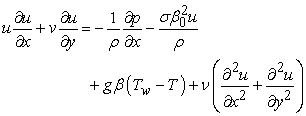(1)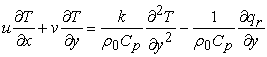(2)

where u and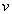are the velocity of the fluid, T is the fluid temperature,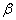is the thermal expansion of the fluid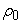is the fluid density,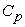is the specific heat capacity, v is the viscosity of the fluid and k is the thermal conductivity

In this research work the mathematical formulation has Magnetic field which is not included in the work of Ibrahim (1997) where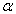the thermal diffusivity andis the radiative, using the Rosseland differential approximation.(3)

The boundary conditions are;(4)

On introducing the following non-dimensional quantities(5)

Substituting the non-dimensional quantities of equation (5) into (1) to (2), leads to(6)(7)

WhereEquation (6) and (7) leads to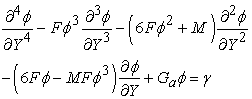(8)(8a)

### 3. Solution to the Problem

To solve equations (8), subjected to the boundary conditions of (8a), the solutions are obtained for temperature and velocity flow. using the Newton scheme method from Taylor series to solve the fourth order non linear problem.(9)

Thus from (8) we have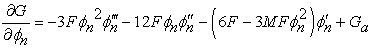(10)(11)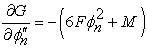(12)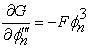(13)(14)

Substituting equations (10) to (14) into equation (9) we obtain(15)

Expanding equations (15) where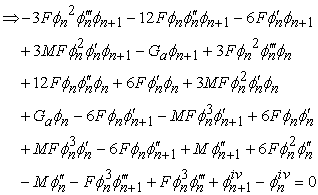(16)

Collecting the terms involvingOn the L.H.S and the terms involvingOn the R.H.S gives(17)

Where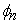is our initial solution andis the assumed solution.

LetConsidering N=6(18)

Putting (18) into (17)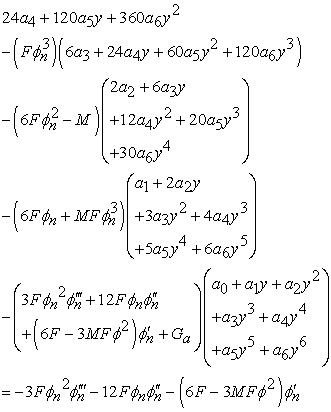(19)

Simplifying by collecting terms in a0, a1, a2, a3, a4, a5, and a6 we obtain.

We now collate equations (20) at point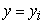, where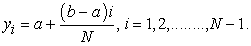In the problem under consideration, N=6, a =-1 and b=+1.

The boundary conditions areThus, we obtain five equations from (20).

Letbe our initial solution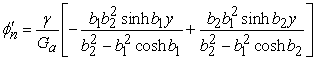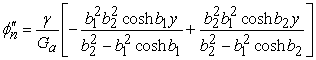We now substitute for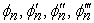into (20) and then solve the equation simultaneously to obtain the unknowns a0, a1, a2, a3, a4, a5, a6 andusing Gaussian elimination method with partial pivoting using MATLAB software to obtain the unknown constants.

But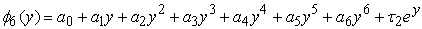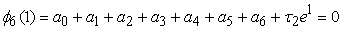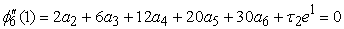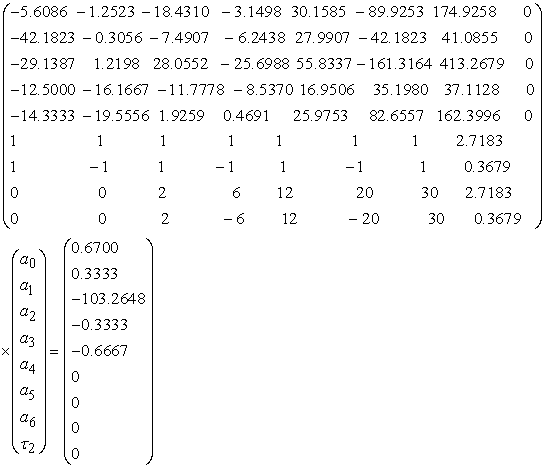(21)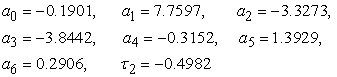Determination of the Temperature profile, to obtain the solution for the temperaturein the interval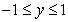since the various values of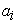and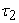are known from the MatLab Program

3.1. Determination of the Velocity Profile

The velocity distribution of the flow can be obtained from equation (6)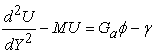(22)(23)

We have our assumed solution to be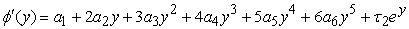Substituting the expression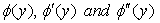into (6) gives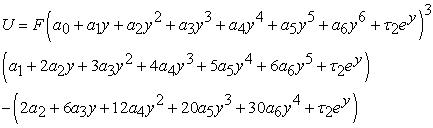(24)

Evaluating equation (6) in the intervalwill enable us to determine the effect of different radiation parameter.

3.2. Determination of the Non-dimensional Flow Rate

The non-dimensional flow rate through the channel per unit width is given by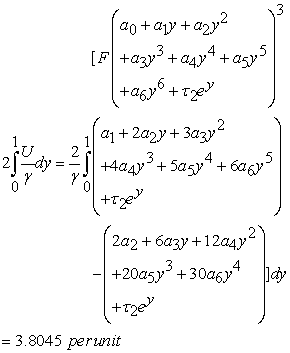(25)

Using the equation (6) and evaluating the integral will enable us to determine the flow rate.

3.3. Determination of Heat Transfer Coefficient

Thus heat transfer coefficient due to thermal conduction is given by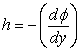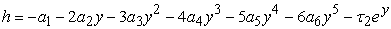(26)

### 4. Results and Discussion

The problem of radiation effect on MHD fluid flow in a vertical channel under optically thick approximation. formulated, analysed and solved numerically. In order to point out the effects of physical parameters namely; thermal Grashof number Ga, radiation parameter F, Magnetic parameter M. on the flow patterns, the computation of the flow fields are carried out. The values of velocity and temperature are obtained for the physical parameters as mention.

The velocity profiles has been studied and presented in Figure 1 to 3 The effect of velocity for different values of Magnetic parameter (M = 5, 10, 15, 20) is presented in Figure 1. The trend shows that the velocity increases with increasing Magnetic parameter. The effect of velocity for different values of radiation (F = 2, 10, 15, 20) is also presented in Figure 2. It is then observed that the velocity decreases with increasing values of radiation. The effect of velocity for different values of thermal Grashof number (Ga= 0.5, 1, 2) is also presented in figure 3. It is then observed that the velocity decreases with increasing values of thermal Grashof number.

Figure 5. Trend of heat transfer coefficient due to thermal conduction h

In Figure 4 It is observed that temperature rise with increasing values ofwhile Figure 5 shows that trend of heat transfer coefficient due to thermal conduction h increases with the rate of change of.

### 5. Summary and Conclusion

Radiation effect on MHD fluid flow in a vertical channel under optically thick approximation has been studied. .In order to point out the effects of physical parameters namely; thermal Grashof number Ga, radiation parameter F, Magnetic parameter M. are presented graphically. It is observed that the velocity increases with increasing Magnetic parameter, while radiation and thermal Grashof number decreases with increasing values of radiation and thermal Grashof number respectively.

It also observed that temperature rise with increasing values ofand trend of heat transfer coefficient due to thermal conduction h increases with the rate of change of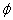.

### References

  Abel, S., K. V. Prasad, and Mahaboob, A.: ‘‘Buoyancy force and thermal radiation effects in MHD boundary layer visco-elastic fluid flow over continuously moving stretching surface,’’ International Journal of Thermal Sciences, vol. 44, no. 5, pp. 465-476. 2005.In article CrossRef  Bharti RP, Chhabra RP, Eswaran V: ‘‘Steady flow of power-law fluids across a circular cylinder,’’ Can. J. Chem. Eng. 84(4) (in press) 2006.In article  Cogley, A. C. L., Vincenti, W. G., Gilles, E. S : ‘‘Differential approximation for radiative heat transfer in a non grey gas near equilibrium,’’ Am. Inst. Aeronat.Astronaut. J 6: 551-553. 1968.In article CrossRef  Crammer, K., Pai, S. I: ‘‘Magnetofluid dynamics for engineers and applied physicists,’’ McGraw-Hill Book Company. 1973.In article  Grief, R., Habib I. S. and Lin J.C.: ‘‘Laminar convection of a radiating gas in a vertical Channel,’’ Journal of Fluid Mechanics Vol. 46, pp 513-522. 1977.In article CrossRef  Ibrahim M.O. ; ‘‘Radiation effect on laminar convective flow of a radiating gas in a vertical channel under optically thick limit approximation’’’ M.Sc thesis , University of Ilorin, Nigeria. 1997.In article  Kearsley, A. J.: ‘‘A steady state model of Couette flow with viscous heating,’’ Int. J. Engng Sci. 32: 179-186. 1994.In article CrossRef  Makinde, O. D., Mhone, P. Y. ‘‘Heat transfer to MHD oscillatory flow in a channel filled with porous medium,’’ Romanian J. Physics 50 (9-10): 931-938. 2005.In article  Makinde, O. D., Osalusi, E (2006), MHD steady flow in a channel with slip at permeable boundaries, Romanian J. Physics 51(3-4): 319-328.In article  Moreau, R.: ‘‘Magnetohydrodynamics,’’ Kluwer Academic Publishers. 1990.In article CrossRef  Taiwo O.A. and Ogunlaran O.M.: ‘‘Numerical solution of fourth order linear ordinary differential equations by cubic spline collocation tau method,’’ Journal of Mathematics and Statistics 4 (4): 264-268, 2008.In article